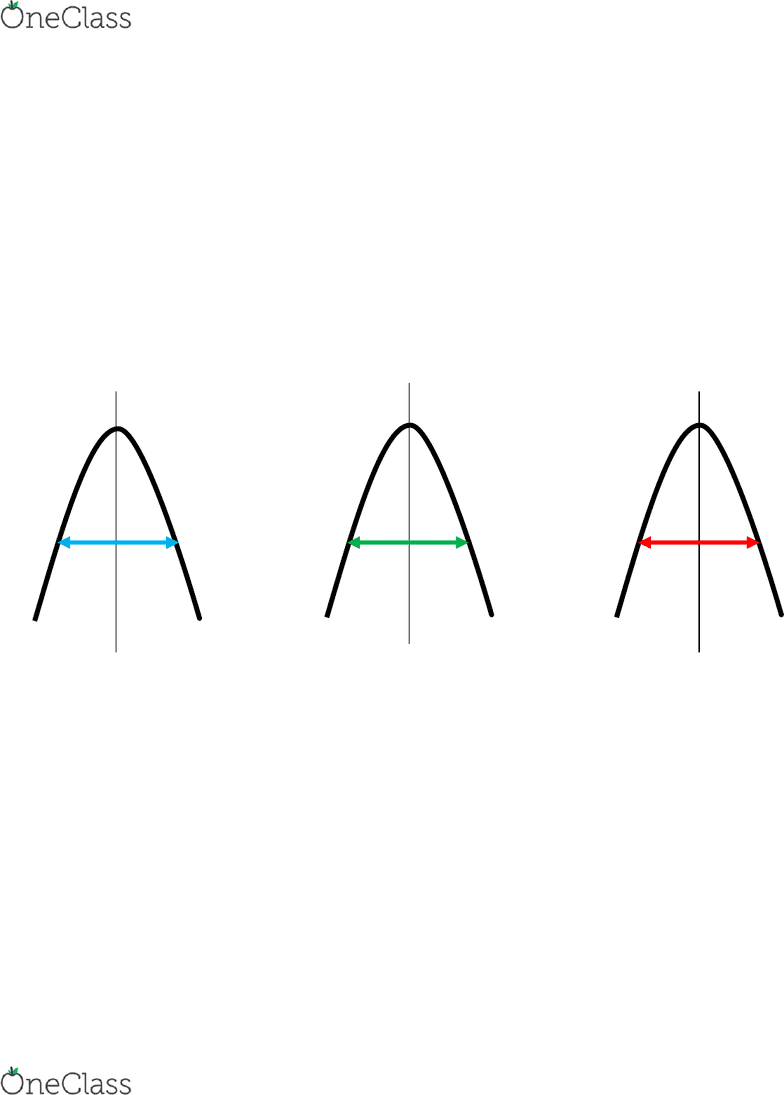# CRIM 320 Lecture Notes - Lecture 7: Multiple Comparisons Problem, Standard Deviation, Confidence Interval

129 views6 pages
School
Department
Course
ProfessorCrim 320 Module 7 Analysis of Variance
- ANOVA = analysis of variance
- ANOVA best thought of as an extension of T-tests
- Like T-tests, ANOVA starts by comparing differences in means between groups
- What happens when your grouping variable has more than two categories
o > 2 groups
- focus on one-way ANOVA
The Logic of ANOVA
- within-group variability
o spread around the mean (variances)
- between-group variability
o differences in group means
- based on these two estimates of variability, you can draw conclusions about the
population means
differences in means does that represent real group differences in the population
Procedure
- first question: are there differences in means across the groups?
o Usually there is some difference in means
- Chance: are observed differences attributable to random variability in the sample?
- OR
- True Difference: Is there reason to believe that some of the groups have different
values in the population?
Hypothesis Testing
- Null hypothesis the population means for all groups is the same
o Aka: there is no differences between group mean scores on the dependent
variable
- Alternative hypothesis: there are differences between groups
Mean
Mean
Mean
find more resources at oneclass.com
find more resources at oneclass.com
Unlock document

This preview shows pages 1-2 of the document.
Unlock all 6 pages and 3 million more documents.- Note: The alternative hypothesis does not state which group differences are
significant
o Because there are more than two groups
One-Way ANOVA
- called one-way because it utilizes one grouping variable
- variable used to form groups is often referred to a factor
- what level of measurement will factors normally be?
o Grouping variable : creating GROUPS = nominal
- What level of measurement do you expect for the dependent variable?
o Interval : comparing means
Assumptions
1) DV is normally distributed for each of the populations as defined by the different
levels of the factor
a. Normal distribution for ALL groups
2) Variances of the DV are the same for all populations
a. Equal variances
b. Requires using Levens Test
3) Random sample
4) Independent of one another
How does ANOVA test for group differences?
- calculate the F ratio
- F = between-groups mean square / within-groups mean square
- Must calculate the between- and within-group variability (mean squares)
Estimating within-group variability I
1) compute within-groups sum of squares
a. obtain variance for each group (SD squared)
i. standard deviation squared
b. multiply each variance by (Group N -1)
c. sum up
2) Compute the degrees of freedom
a. Sum of (Group N-1) for all groups
i. There are 2 df
3) Finally, divide the sum of squares by its degrees of freedom
4) WMS Within Mean Square
a. Average of the variances in each of the groups, adjusted for the number of
observations in each group
Estimating Between-group Variability I
1) compute between-groups sum of squares
a. subtract overall mean from each group mean
b. square each of these differences
c. multiply the square by number in each group
d. sum up
2) compute the degrees of freedom (df)
a. number of groups -1
3) divide the sum of squares by df (step1 / step2)
4) BMS Between mean square
find more resources at oneclass.com
find more resources at oneclass.com
Unlock document

This preview shows pages 1-2 of the document.
Unlock all 6 pages and 3 million more documents.

# Get access

\$10 USD/m
Billed \$120 USD annually
Homework Help
Class Notes
Textbook Notes